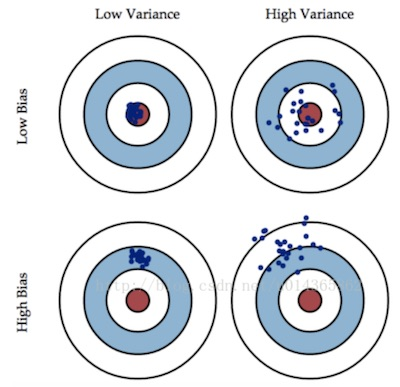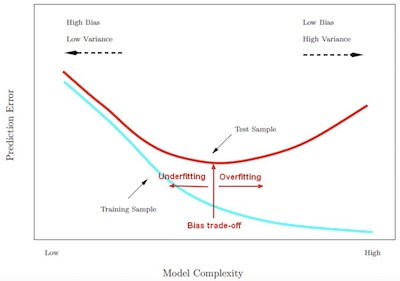### Why should I choose AnalystNotes?

Simply put: AnalystNotes offers the best value and the best product available to help you pass your exams.

##### Subject 2. Overview of Evaluating ML Algorithm Performance
Overfitting occurs when a model tries to fit the training data so closely that it does not generalize well to new data.

Generalization refers to the model's ability to adapt properly to new, previously unseen data, drawn from the same distribution as the one used to create the model.

There are three types of samples:
1. training sample: The subset of the data set used to train a model.
2. validation set: A subset of the data set - disjunct from the training set - that you use to validate the model and adjust�hyperparameters.
3. test set: The subset of the data set that you use to test your�model�after the model has gone through initial vetting by the validation set.
Overfitting means low error on training data and high error on test data.

• It is when you create a model which is predicting the noise in the data rather than the real signal.
• Overfitting generally occurs when a model is excessively complex, such as having too many parameters relative to the number of observations.

#### Errors and Overfitting

In machine learning, the bias-variance tradeoff (or dilemma) is the problem of simultaneously minimizing two sources of error that prevent supervised learning algorithms from generalizing beyond their training set:

• The bias is error from erroneous assumptions in the learning algorithm. High bias can cause an algorithm to miss the relevant relations between features and target outputs (underfitting).
• The variance is error from sensitivity to small fluctuations in the training set. High variance can cause overfitting: modeling the random noise in the training data, rather than the intended outputs.The bias-variance decomposition is a way of analyzing a learning algorithm's expected generalization error with respect to a particular problem as a sum of three terms, the bias, variance, and a quantity called the base error (irreducible), resulting from noise in the problem itself.

Eout = bias error + variance error + base error
The bias-variance trade-off is the point where we are adding just noise by adding model complexity (flexibility). The training error (bias error) goes down as it has to, but the test error (variance error) is starting to go up. The model after the bias-variance trade-off begins to overfit.#### Preventing Overfitting

There are two common principles:
1. Complexity reduction: simple algorithms, limit the number of features, etc to keep things simple.
2. Avoid sampling bias: K-fold-cross-validation technique can be used to mitigate the holdout sample problem (excessive reduction of the training set size).